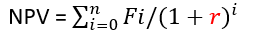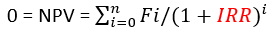# Decision Making TechniquesDecision making techniques lead organisations in the right direction when making critical decisions or planning for the future. Generally, decision making is an essential part of life. There are various approaches to decision making that apply to different kinds of situations. Below are the major financial decision-making techniques and general decision-making techniques that organisations can apply in order to make the best possible choices.

## Financial Decision-Making Techniques

Financial managers make decisions in order to maximise the profitability of the firm. These involve financing, capital budgeting and investment decisions. There are different kinds of financial decision-making techniques that can be used by these managers. The most common ones are, cost benefit analysis, net present value and break-even analysis to name a few.

### Cost Benefit Analysis (CBA)

One of the most effective financial decision-making tools is the cost benefit analysis (CBA) also known as the benefit cost analysis. This technique is a quick and simple technique used for non-critical financial decision making. A cost benefit analysis assesses the costs and benefits associated with a course of action.  For example, a firm may asses the costs and benefits of purchasing a new delivery van. If the benefits outweigh the costs, then the purchase can be made but if costs exceed the benefits the purchase will not be made.

### Break Even Analysis

A break-even analysis is an essential decision-making technique. It is used to determine the output at which the income generated by an investment equals the costs associated with it. At breakeven, the revenue generated equals the total costs, that is, at that output no profit or loss is made. If more output is produced beyond that point, profit can be obtained and if less output is produced below that point, the firm will make a loss. With this financial decision-making technique, a company can project the profits or losses that can occur at any given quantity. In addition, a breakeven analysis can be used to set the right price to avoid making a loss and to assess the effects of an increase or a decrease in costs.

### The Net Present Value (NPV)

When an organisation needs to determine if it is profitable to invest in a new plant and machinery or introduce a new product, the net present value (NPV) decision making technique can be applied. The net present value is the difference between discounted future cash inflows and cash outflows associated with a given investment over a specific period of time. If the net present value is positive an investment in the project will be approved but if the net present value is negative the firm will not undertake the investment.Where Fi = net cash flow

r = the discount rate

i = time/periods

### Internal Rate of Return (IRR)

The Internal rate of return (IRR) is another financial decision-making technique. IRR is a measure of the rate of return from an investment. The internal rate of return is an estimate of an investment’s break-even discount rate of return which is used to indicate an investment’s profitability. This means that IRR is the discount rate where the net cashflow of a given investment is zero. The rate at which the sum of all cash inflows and outflows equal zero. If the IRR of a new project exceeds the company’s required rate of return then the project will be undertaken, if not, the project will be rejected.  The required rate of return that is used to compare IRR is the discount rate that is used in calculating the NPV. This discount rate can be referred to as an opportunity cost.If IRR is greater than r (from the NPV formula) then the project will be undertaken

It is important to note that the value for IRR cannot be determined algebraically, it must be found by mathematical trial and error.

## General Models of Decision Making

In addition to financial decision-making techniques, there are other techniques that managers and employees can make use of with regards to major projects and other important decisions.

### The Kepner- Tregoe Matrix

General decision making in business can be guided by decision making techniques such as the Kepner-Tregoe Matrix. This technique helps sort out all the important factors before making the final choice. In applying this technique, these four steps should be followed

1. First, a situational analysis, where everything that is involved in a choice is assessed at a broader level.
2. Problem analysis, the exact problem or issue is evaluated.
3. Decision analysis, an identification or evaluation of various alternatives by performing a risk analysis for each possible choice.
4. Potential problem analysis, this is when the final decision and the risks associated with it are evaluated so that preventive actions necessary to minimise the risks are taken.

### The future’s wheel

This is a practical decision-making technique which is still in use from the time it was introduced in the 70’s. The future’s wheel helps organisations determine if they are headed in the right direction. The future is uncertain, and the environment can change at any time. This may be a result of changing market forces or a change from within the organisation. In order to apply the future’s wheel, the major change, objective or goal is written down at the centre, from there supporting activities that need to be implemented in order to achieve the main goal are added. Deeper levels of change are added with the spokes getting longer, that way a wheel is gradually created.

### A Force Field Analysis

The force field analysis decision-making technique is simple and effective. it helps in accurately assessing the forces that may work against a plan or that may work in favour of it. It requires one to write down a clearly defined plan with two columns. One column containing factors that might complement a plan and the other column, factors that may threaten it, with each factor assigned a value based on the positive or negative impact. Lastly, the negative and positive factor values are added so that one can see if the plan is viable or not. If the total value for the negative factors is high, pursuing the plan might not be advisable but if the sum for the positive factors is higher than the negative factors, then the plan should be considered.